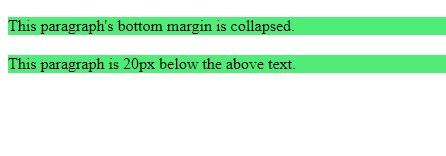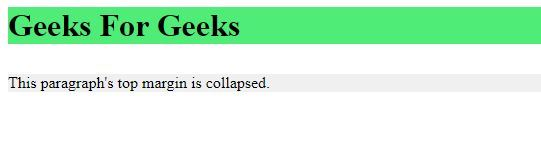Open in App
Not now

# CSS Margin Collapse

• Difficulty Level : Expert
• Last Updated : 02 Jul, 2021

The CSS margin property is used to set a margin on all four sides of an element.
When two elements are next to each other on a page vertically, one of the elements might lose its vertical margin. This means the top and bottom margins of elements are sometimes combined into a single margin. The single margin is equal to the largest of the two margins getting collapsed.
Example:

## html

 `````<``html` `lang``=``"en"` `dir``=``"ltr"``>``    ``<``head``>``       ``<``style``>``           ``p{``               ``margin: 20px 0 10px;``               ``background-color:rgb(79, 236, 119);``           ``}``       ````    ````    ``<``body``>``        ` `<``p``>This paragraph's bottom margin is collapsed.` `        ` `<``p``>This paragraph is 20px below the above text.` `    `````

You would expect the margin between the two paragraphs to be 30px (10px bottom margin of 1st paragraph + 20px top margin of next paragraph). But in CSS the bigger margin overrides and the actual margin is the bigger one (20px).
Output:If one element has a negative margin, the negative margin is subtracted from the positive margin. For example, resultant margin of 40px and -30px will be 10px (40px-30px). If both the margins are negative, greater negative value is used. For example, resultant margin of -20px and -50px will be -50px.
The margins of floating and absolutely positioned elements never collapse.
Some specific cases of margins collapse:

The margins of vertically adjacent elements collapse as we saw in the previous example.
• Between parent and child elements:
Adjacent margins of parent and child elements collapse when no padding, borders, or content exist between the adjacent margins.

Example:

## html

 ```<``html` `lang``=``"en"` `dir``=``"ltr"``>``    ``<``head``>``       ``<``style``>``           ``h1{``              ``margin: 0;``              ``background-color: rgb(79, 236, 119);``           ``}` `           ``div{``               ``margin: 30px 0 20px ;``           ``}` `           ``p{``               ``margin: 20px 0 10px;``               ``background-color: #f0f0f0;``           ``}``       ````    ````    ``<``body``>``        ``<``h1``>Geeks For Geeks``        ``<``div``>``            ` `<``p``>This paragraph's top``              ``margin is collapsed.` `        ````    `````

Output:Here the margin between h1 and div is 30px and not 50px (30px + 20px).

Supported Browsers: